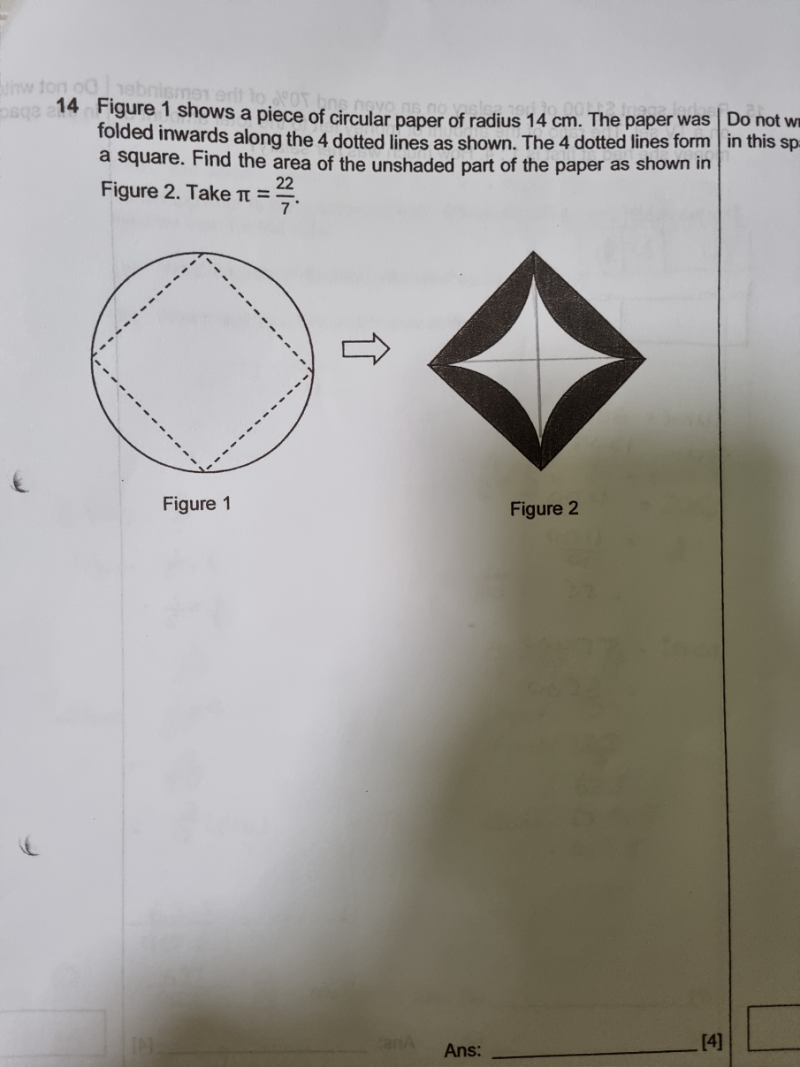# QuestionThank you

Alternative Solution:

The square is made up of 4 right-angled triangles.

Radius of circle = 14cm = base = height of each right-angled triangle

Hence area of square = 4  x [ (1/2) x 14 x 14 ] = 392

Area of circle = (22/7) x 14 x 14= 616

Area of 4 chords = 616-392 = 224

Hence Area of unshaded part = Area of square – Area of 4 chords = 392-224 = 168 cm2

0 Replies 1 Like

Diameter of circle = 14+14 = 28cm

Using Pythagoras Theorem,
(side of sq)^2 + (side of sq)^2 = (28)^2
2 (side of sq)^2 = (28)^2
(side of sq)^2 = 392
(side of sq)^2 = Area of square = 392cm^2

Area of circle = 22/7 * 14^2 = 616cm^2

616cm^2- 392 = 224cm^2 (Shaded part of circle)

Area of square – (Shaded part of circle folded in)
= 392cm^2 – 224 cm^2
= 168cm^2 (area of unshaded part of paper)

0 Replies 1 Like